[Next]: Stress analysis of thin wafers
 [Up]: Project descriptions
 [Previous]: Wave propagation in porous and granular
[Contents]   [Index]

## Thermodynamic modeling of porous and granular materials

Collaborator: K. Wilmanski

Cooperation with: I. Müller (Technische Universität (TU) Berlin), R. Lancellotta (Università di Torino, Italy), C. Lai (Studio Geotecnico Italiano, Milano)

Description: Aims and results of the project

The main task in this project is to establish a thermodynamic framework for multicomponent nonlinear thermomechanical models of porous materials. Due to the choice of the skeleton as the reference, the so-called Lagrangian description of motion  is used. Consequently, if we consider a porous medium whose channels are filled with a mixture of A fluid components, the model is constructed on a chosen reference configuration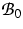of the solid component, i.e. all fields are functions of a spatial variable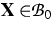, and time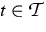. In the work  we consider a thermomechanical model in which the governing fields are as follows:

1.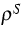- mass density of the skeleton in the reference configuration;
2.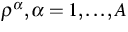- partial mass densities of fluid components referring to the unit volume of the reference configuration of the skeleton;

3.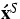- velocity field of the skeleton;

4.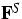- deformation gradient of the skeleton;

5.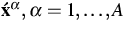- velocity fields of fluid components;

6.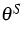- absolute temperature of the skeleton;

7.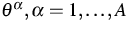- absolute temperatures of fluid components;

8.
n - porosity (the volume fraction of voids).

Such a model was indicated in the work  (see as well [3, 4]), but the results were primarily related to the hierarchic structure of the so-called extended thermodynamics. In the present work we are primarily concerned with similarities and differences of the model with the classical Truesdell model of fluid mixtures. We show that the barycentric velocity of this classical theory must be replaced by the following relative Lagrangian velocity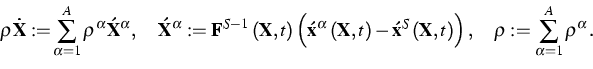With this relation in mind we obtain the following relation for the bulk Piola-Kirchhoff stress tensor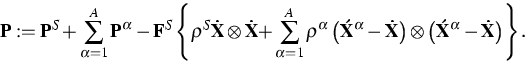This formula has a formal similarity to the classical Truesdell relations but it refers to the skeleton rather than to the center of gravity, and it is in the Lagrangian rather than Eulerian form.

Similarly we obtain for the bulk internal energy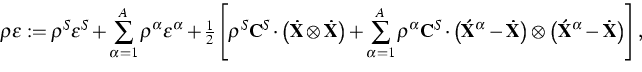where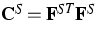is the Cauchy-Green deformation tensor for the skeleton, and for the bulk heat flux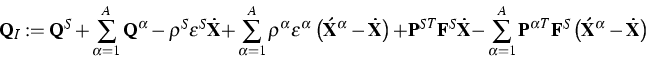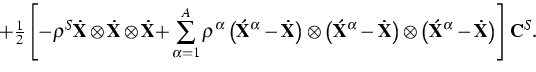These relations possess again a formal similarity to the Truesdell relations with the difference described above for the stress tensor.

By means of these relations one can evaluate the second law of thermodynamics which is assumed to have the form proposed by I. Müller (TU Berlin) for mixtures of fluids. In the Lagrangian form it is as follows. The entropy inequality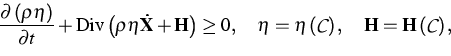where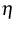is the entropy density in the reference configuration,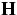is the bulk entropy flux, and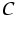denotes the set of constitutive variables, must hold for all solutions of field equations. An evaluation of this law has been done for fully nonlinear poroelastic isotropic materials with ideal fluid components. We discuss in detail the two-component case which has been investigated already in the projects of the previous years.

References:

1. K. WILMANSKI, Lagrangian model of two-phase porous material, J. Non-Equilibrium Thermodyn., 20 (1995), pp. 50-77.
2., Towards an extended thermodynamics of porous and granular materials, in: Trends in Applications of Mathematics to Mechanics, G. Iooss, O. Guès, A. Nouri, eds., Monographs and Surveys in Pure and Applied Mathematics, 106, Chapman & Hall/CRC, Boca Raton, 2000, pp. 147-160.
3., Thermodynamics of multicomponent continua, in: Earthquake Thermodynamics and Phase Transformations in the Earth's Interior, R. Teisseyre, E. Majewski, eds., International Geophysics Series, 76, Academic Press, San Diego, 2001, chapter 25, pp. 567-656.
4., Mass exchange, diffusion and large deformations of poroelastic materials, in: Modeling and Mechanics of Granular and Porous Materials, G. Capriz, V.N. Ghionna, P. Giovine, eds., Model. Simul. Sci. Engrg. Technol., Birkhäuser, Boston, 2003, pp. 211-242.
5., On thermodynamics of nonlinear poroelastic materials, WIAS Preprint no. 792, 2002, to appear in: J. Elasticity.

 [Next]: Stress analysis of thin wafers
 [Up]: Project descriptions
 [Previous]: Wave propagation in porous and granular
[Contents]   [Index]

LaTeX typesetting by I. Bremer
5/16/2003